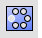# ArrayHoleSolid

Solid Edit Tools >

Holes >

Array Hole

The ArrayHole command copies holes in a surface in a specified number rows and columns.

#### Steps

1. Select a hole.
2. Type the number of holes in the A direction.
3. Type the number of holes in the B direction.
4. Pick a base point.
5. Pick the A direction and distance.
6. Pick the B direction and distance.
Command-line options

Changes the first direction.

ANumber

Number of holes in the first direction.

ASpacing

Distance between hole centers in the first direction.

BNumber

Number of holes in the second direction.

BSpacing

Distance between hole centers in the second direction.

Rectangular

Forces the second direction to be perpendicular to the first direction.

UseASpacing

Use the same distance as the second direction as for the first direction.

# ArrayHolePolarSolid

Solid Edit Tools >

Holes >

Array Hole Polar

The ArrayHolePolar command copies holes around a central location.

#### Steps

1. Select a hole.
2. Pick a Center for the array.
The rotation axis for the polar array is the normal direction of the surface.
3. Type the number of holes.
4. Type an angle to fill, and press Enter.
Command-line options

Angle

The number of degrees of a circle to fill.

Number

The number of holes in the array.